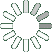Puzzle Command
Daily Puzzle
Number Logic #2
November 29, 2014Find a 6 digit number where:

• The 1st digit is 1.
• The 2nd digit is 2.
• The 3rd digit is 3.
• The 4th digit is the number of 2s in the number.
• The 5th digit is the sum of the 1st, 2nd, 3rd, and 4th number.
• The 6th digit is the sum of the 4th and 5th number.
Puzzle Notes
The 1st digit is 1.
The 2nd digit is 2.
The 3rd digit is 3.
The 4th digit is the number of 2s in the number.
The 5th digit is the sum of the 1st, 2nd, 3rd, and 4th number.
The 6th digit is the sum of the 4th and 5th number.
Enter in your solution below and click submit.